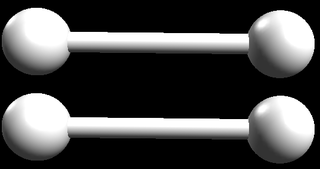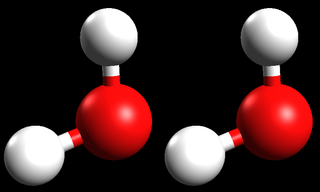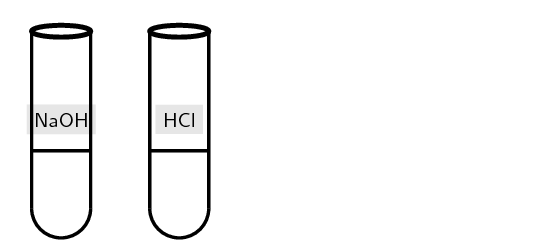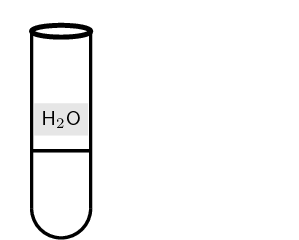Home Practice
For learners and parents For teachers and schools
Textbooks
Full catalogue
Pricing SupportLog in

We think you are located in United States. Is this correct?

# 13.2 Conservation of atoms and mass in reactions

## 13.2 Conservation of atoms and mass in reactions (ESADV)

In a chemical reaction the total mass of all the substances taking part in the reaction remains the same. Also, the number of atoms in a reaction remains the same. Mass cannot be created or destroyed in a chemical reaction.

Law of conservation of mass

The law of conservation of mass states that the total mass of substances taking part in a chemical reaction is conserved during the reaction.

Table 13.1 illustrates this law for the decomposition of hydrogen peroxide.

## The conservation of atoms in chemical reactions

We will use the reaction of hydrogen and oxygen to form water in this activity.

### Materials

Coloured modelling clay rolled into balls or marbles and prestik to represent atoms. Each colour will represent a different element.

### Method

1. Build your reactants. Use marbles and prestik or modelling clay to represent the reactants and put these on one side of your table. Make at least ten ($$\text{H}_{2}$$) units and at least five ($$\text{O}_{2}$$) units.

2. Place the $$\text{H}_{2}$$ and $$\text{O}_{2}$$ units on a table. The table represents the “test tube” where the reaction is going to take place.

3. Now count the number of atoms ($$\text{H}$$ and $$\text{O}$$) you have in your “test tube”. Fill in the reactants column in the table below. Refer to Table 13.1 to help you fill in the mass row.

4. Let the reaction take place. Each person can now take the $$\text{H}$$ and $$\text{O}$$ unit and use them to make water units. Break the $$\text{H}$$ and $$\text{O}$$ units apart and build $$\text{H}_{2}\text{O}$$ units with the parts. These are the products. Place the products on the table.

5. When the “reaction” has finished (i.e. when all the $$\text{H}$$ and $$\text{O}$$ units have been used) count the number of atoms ($$\text{H}$$ and $$\text{O}$$) and complete the table.

6. What do you notice about the number of atoms for the reactants, compared to the products?

7. Write a balanced equation for this reaction and use your models to build this equation.

 Reactants ProductsNumber of molecules Mass Number of atoms

### Discussion

You should have noticed that the number of atoms in the reactants is the same as the number of atoms in the product. The number of atoms is conserved during the reaction. However, you will also see that the number of molecules in the reactants and products are not the same. The number of molecules is not conserved during the reaction.

## Conservation of matter

### Aim

To prove the law of conservation of matter experimentally.

### Materials

Reaction 1:

3 beakers; silver nitrate; sodium iodide; mass meter

Reaction 2:

hydrochloric acid; bromothymol blue; sodium hydroxide solution; mass meter

Reaction 3:

any effervescent tablet (e.g. Cal-C-Vita tablet), balloon; rubber band; mass meter; test tube; beaker

Always be careful when handling chemicals (particularly strong acids like hydrochloric acid) as you can burn yourself badly.

### Method

Reaction 1

1. Solution 1: In one of the beakers dissolve $$\text{5}$$ $$\text{g}$$ of silver nitrate in $$\text{100}$$ $$\text{mL}$$ of water.

2. Solution 2: In a second beaker, dissolve $$\text{4,5}$$ $$\text{g}$$ of sodium iodide in $$\text{100}$$ $$\text{mL}$$ of water.

3. Determine the mass of each of the reactants.

4. Add solution 1 to solution 2. What do you observe? Has a chemical reaction taken place?

5. Determine the mass of the products.

6. What do you notice about the masses?

7. Write a balanced equation for this reaction.Reaction 2:

1. Solution 1: Dissolve $$\text{0,4}$$ $$\text{g}$$ of sodium hydroxide in $$\text{100}$$ $$\text{mL}$$ of water. Add a few drops of bromothymol blue indicator to the solution.

2. Solution 2: Measure $$\text{100}$$ $$\text{mL}$$ of $$\text{0,1}$$ $$\text{mol·dm^{-3}}$$ hydrochloric acid solution into a second beaker.

3. Determine the mass of the reactants.

4. Add small quantities of solution 2 to solution 1 (you can use a plastic pipette for this) until a colour change has taken place. Has a chemical reaction taken place?

5. Determine the mass of hydrochloric acid added. (You do this by weighing the remaining solution and subtracting this from the starting mass)

6. Compare the mass before the reaction to the total mass after the reaction. What do you notice?

7. Write a balanced equation for this reaction.Reaction 3

1. Half fill a large test tube with water.

2. Determine the mass of the test tube and water.

3. Break an effervescent tablet in two or three pieces and place them in a balloon.

4. Determine the mass of the balloon and tablet.

5. Fit the balloon tightly to the test tube, being careful to not drop the contents into the water. You can stand the test tube in a beaker to help you do this.

6. Determine the total mass of the test tube and balloon.

7. Lift the balloon so that the tablet goes into the water. What do you observe? Has a chemical reaction taken place?

8. Determine the mass of the test tube balloon combination.

9. What do you observe about the masses before and after the reaction?### Results

Fill in the following table for the total mass of reactants (starting materials) and products (ending materials).

 Reaction 1 Reaction 2 Reaction 3 Reactants Products

Add the masses for the reactants for each reaction. Do the same for the products. For each reaction compare the mass of the reactants to the mass of the products. What do you notice? Is the mass conserved?

In the experiment above you should have found that the total mass at the start of the reaction is the same as the mass at the end of the reaction. Mass does not appear or disappear in chemical reactions. Mass is conserved, in other words, the total mass you start with is the total mass you will end with.

## Conservation of atoms and mass

Textbook Exercise 13.2

Complete the following chemical reactions to show that atoms and mass are conserved. For each reaction give the total molecular mass of the reactants and the products.

Hydrogen gas combines with nitrogen gas to form ammonia.

Solution not yet available

Hydrogen peroxide decomposes (breaks down) to form hydrogen and oxygen.

Solution not yet available

Calcium and oxygen gas react to form calcium oxide.

Solution not yet available